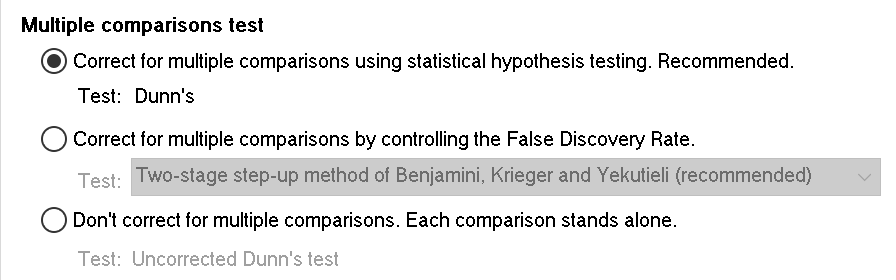## Please enable JavaScript to view this site.

 How the Dunn method for nonparametric comparisons works

## Two forms of the Dunn's test

On Prism's option tab of the parameters dialog, you can choose two different form of Dunn's test.Prism performs the Dunn's multiple comparison test(1), which is standard. One source is Daniel's Applied nonparametric statistics, second edition page 240-241. Some books and programs don't use Dunn's name, but simply refer to this test as the post test following a Kruskal-Wallis test, and don't give it an exact name.

## Step 1. Calculate z

For ordinary (not matched, not repeated measures) nonparametric ANOVA: To compare group i and j, find the absolute value of the difference between the mean rank of group i and the mean rank of group j. If there are no ties, compute z by dividing this difference in mean ranks by the square root of [(N*(N+1)/12)*(1/Ni + 1/Nj)]. Here N is the total number of data points in all groups, and Ni and Nj are the number of data points in the two groups being compared. If there are ties, calculate z by dividing the difference in mean ranks by the square root of [(N*(N+1) - Sum(Ti^3 - Ti) / (N - 1)) / 12 * (1/Ni + 1/Nj), where Ti is the number of ties in the i-th group of ties.

For repeated measures nonparametric ANOVA (Friedman's test): To compare treatment i and j, find the absolute value of the difference between the mean rank of group i and the mean rank of group j. Calculate z by dividing this difference in mean ranks by the square root of [K(K+1)/(6N)]. Here N is the number of matched sets of data, which is the number of rows in the data table, and K is the number of treatment groups (number of columns).

## Step 2. Calculate the uncorrected P value

Calculate the two-tail (two-sided) P value that corresponds to the z ratio you just calculated. This free web calculator can do the job.

If you chose the uncorrected Dunn's test, you are done. Compare the P value to alpha to decide whether or not the result is statistically significant.

## Step 3. If you want to correct for multiple comparisons,, compute the multiplicity adjusted P value

Multiply the uncorrected P value computed in step 2 by K. If this product is less than 1.0, it is the multiplicity adjusted P value. If the product is greater than 1.0 the multiplicity adjusted P value is reported as > 0.9999

## Compare the multiplicity adjusted P value to alpha to decide whether or not the result is statistically significant.

Notes

When comparing two groups as part of Dunn's test, the ranks are those for all the values. Rank all the values, compute the mean rank in each group, and do the calculations above. Don't create ranks just for the values in those two groups.

This method accounts for ties when computing the ranks, and thus when computing the mean ranks which are compared.

It would seem sensible to base multiple comparisons tests after nonparametric ANOVA to be based on the Mann-Whitney or Wilcoxon method to compute P values, followed by Bonferroni or other method to correct for multiple comparisons. Prism does not offer this approach, because it is not commonly used (but we'd appreciate comments and suggestions).

## Reference

1. O.J. Dunn, Technometrics, 5:241-252, 1964